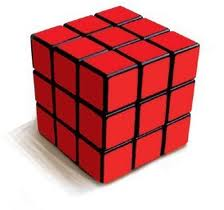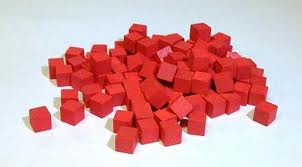The painted cube

Question:You  have 10x10x10 cube which is made up by 1x1x1 smaller size cubes. Imagine a Rubik’s Cube.

The outside of the big cube is painted red on all 6 sides .  If the cube was to be to torn apart to its 1x1x1 smaller cubes, how many of them would have red paint on any of their sides ?Answer:  You know there are 10x10x10=1000 smaller cubes.

Start by finding the ones that are not painted .  Those will be the ones that are not in the outside of the larger cube.   . That will be  (10-2)x(10-2)x(10-2) = 8x8x8= 512 inner cubes .               So the number of painted will be  1000-512=488.

Note: if you are asked a different cube size use  the following formula

X3 – (X-2) 3   where X is the number of smaller cubes each dimention has   .